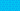PricingResources

# How to calculate the payback period

Written by

Before making any investment decision, it’s helpful to think about how long it will take back to recover your initial cost. This is the basic principle behind the payback period. Learn more about how to calculate payback period, and what it means for your investments, below.

## What does 'payback period' mean?

The payback period is the amount of time it would take for an investor to recover a project's initial cost. It's closely related to the break-even point of an investment.

Payback period is a quick and easy way to assess investment opportunities and risk, but instead of a break-even analysis’s units, payback period is expressed in years. The shorter the payback period, the more attractive the investment would be, because this means it would take less time to break even.

Payback period is used not only in financial industries, but also by businesses to calculate the rate of return on any new asset or technology upgrade. For example, a small business owner could calculate the payback period of installing solar panels to determine if they’re a cost-effective option.

## How to calculate payback period

To determine how to calculate payback period in practice, you simply divide the initial cash outlay of a project by the amount of net cash inflow that the project generates each year. For the purposes of calculating the payback period formula, you can assume that the net cash inflow is the same each year. The resulting number is expressed in years or fractions of years.

## Payback period formula

Written out as a formula, the payback period calculation could also look like this:

For example, imagine a company invests \$200,000 in new manufacturing equipment which results in a positive cash flow of \$50,000 per year.

In this case, the payback period would be 4.0 years because 200,0000 divided by 50,000 is 4.

### Pros of payback period analysis

Acting as a simple risk analysis, the payback period formula is easy to understand. It gives a quick overview of how quickly you can expect to recover your initial investment. The payback period also facilitates side-by-side analysis of two competing projects. If one has a longer payback period than the other, it might not be the better option.

### Cons of payback period analysis

On the other hand, payback period calculations can be so quick and easy that they’re overly simplistic.

One of the disadvantages of this type of analysis is that although it shows the length of time it takes for a return on investment, it doesn’t show the specific profitability. This can be a problem for investors choosing between two projects on the basis of the payback period alone. One project might be paid back faster, but – in the long run – that doesn’t necessarily make it more profitable than the second. Some investments take time to bring in potentially higher cash inflows, but they will be overlooked when using the payback method alone.

Another drawback to the payback period is that it doesn’t take the time value of money into account, unlike the discounted payback period method. This concept states that money would be worth more today than the same amount in the future, due to depreciation and earning potential.

## Alternatives to using payback period calculation

Using the payback period to assess risk is a good starting point, but many investors prefer capital budgeting formulas like net present value (NPV) and internal rate of return (IRR). This is because they factor in the time value of money, working opportunity cost into the formula for a more detailed and accurate assessment. Another option is to use the discounted payback period formula instead, which adds time value of money into the equation.

For the most thorough, balanced look into a project’s risk vs. reward, investors should combine a variety of these models.

### We can help

GoCardless helps businesses automate collection of both regular and one-off payments, while saving time and reducing costs. Find out how GoCardless can help you with ad hoc or recurring payments.

Sales

Support

+61 3 8375 9198